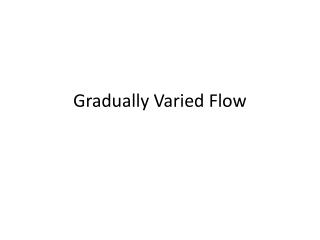DownloadDownload PresentationDownload Presentation- - - - - - - - - - - - - - - - - - - - - - - - - - - E N D - - - - - - - - - - - - - - - - - - - - - - - - - - -
##### Presentation Transcript

2. Basin Assumptions • We consider the flow to be Gradually Varied Flows if the rate of variation of depth with respect to distance is small. • The theory of Gradually Varied Flow is based upon: • The head loess at a section is the same as for a uniform flow having the velocity and hydraulic radius of the section • The slope of the channel is small • The channel is prismatic; that is, the channel has constant alignment and shape • The velocity distribution in the channel section is fixed. • The conveyance factor and section factor are exponential functions of the depth of flow • The roughness coefficient is independent of the depth of flow and constant throughout the channel under construction.

3. Governing Equations • Consider a distance, , as positive in the down streamflow direction. • + • (Eq. 5-7; important in water surface) • The negative sign since both and decrease as increases (Choudhry, 2008)

4. Classification of Water-Surface Profiles • Notation (CDL; NDL) critical depth line; normal depth line • Channel bottom slopes are classified into the following five categories: mild (), steep (), critical (), horizontal () [zero slope] and adverse () [negative slope] • Bottom slope • Mild if ; • Steep if ; and • Critical if • Zone 1: region above both critical and normal lines. Zone 2 between upper and lower lines. Zone 3 between lower line and channel bottom = Steep channel bottom slope at Zone 1 (Choudhry, 2008)

5. Water surface profiles (Choudhry, 2008)

6. Remarks • By determining the sign of the numerator and the denominator of we can make qualitative observations about various types of water surface profiles. • Observations indicate whether the depth increases or decreases with distance, how the profiles end at the upstream and at the downstream limits.

7. Remarks 2 • The flow depth increases with distance if is positive and it decreases if is negative. • By determining the sign of the numerator and the denominator of we can say whether the flow depth for a particular profile increases of decreases with distance.

8. Remarks 3 • As discussed before for a uniform flow when . • From Manning and Chezy Equations for a specified discharge, , • if . • if • Using these inequalities and the Froude number will determine the sign of the numerator and the denominator

9. Types of Flow Profiles (Chow, 1959)

10. Profiles (Chow, 1959)

11. Example • The normal and critical depths of the flow in a channel have been computed and are shown in Figure below. Sketch the possible flow profile.

12. Sketch (Chow, 1959)